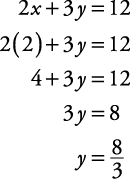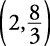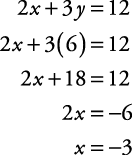## Equations of Lines

Equations involving one or two variables can be graphed on any x–y coordinate plane. In general, it is true that

• if a point lies on the graph of an equation, then its coordinates make the equation a true statement, and

• if the coordinates of a point make an equation a true statement, then the point lies on the graph of the equation.

The graphs of linear equations are always lines. All linear equations can be written in the form Ax + By = C, where A, B, and C are real numbers and A and B are not both zero. Furthermore, to be in standard form, A has to be a positive number. Following are examples of linear equations and their respective A, B, and C values.

x + y = 0

3x – 4y = 9

x = –6

y = 7

A = 1

A = 3

A = 1

A = 0

B = 1

B = –4

B = 0

B = 1

C = 0

C = 9

C = –6

C = 7

Following are terms with which you should be familiar:

• Standard form. The form Ax + By = C for the equation of a line is known as the standard form for the equation of a line.

• x‐intercept. The x‐intercept of a graph is the point at which the graph will intersect the x‐axis. It will always have a y‐coordinate of zero. A horizontal line that is not the x‐axis will have no x‐intercept.

• y‐intercept. The y‐intercept of a graph is the point at which the graph will intersect the y‐axis. It always has an x‐coordinate of zero. A vertical line that is not the y‐axis has no y‐intercept.

One way to graph a linear equation is to find solutions by giving a value to one variable and solving the resulting equation for the other variable. A minimum of two points is necessary to graph a linear equation.

##### Example 1

Draw the graph of 2 x + 3 y = 12 by finding two random points.

To do this, select a value for one variable; then substitute this into the equation and solve for the other variable. Do this a second time with new values to get a second point.

Let x = 2; then find y.Therefore, the ordered pairbelongs on the graph.

Let y = 6; then find x.Therefore, the ordered pair (–3,6) belongs on the graph.

As shown in Figure 1, graph these points and then connect them to make the line that represents the graph of 2 x + 3 y =12.